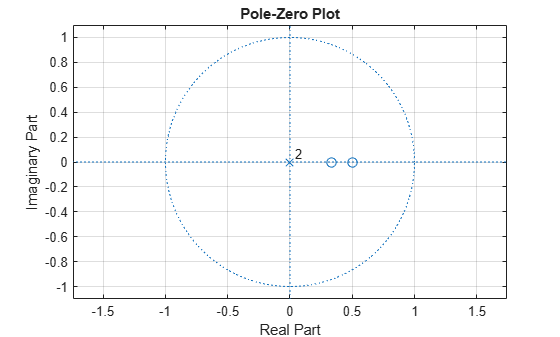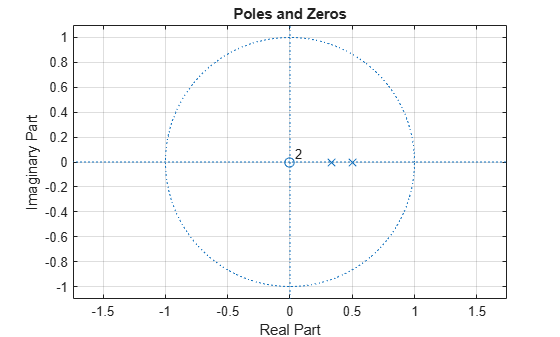Prediction Polynomial

This example shows how to obtain the prediction polynomial from an autocorrelation sequence. The example also shows that the resulting prediction polynomial has an inverse that produces a stable all-pole filter. You can use the all-pole filter to filter a wide-sense stationary white noise sequence to produce a wide-sense stationary autoregressive process.

Create an autocorrelation sequence defined by

$r\left(k\right)=\frac{24}{5}\phantom{\rule{0.16666666666666666em}{0ex}}{2}^{-|k|}-\frac{27}{10}\phantom{\rule{0.16666666666666666em}{0ex}}{3}^{-|k|},\phantom{\rule{1em}{0ex}}k=0,1,2.$

k = 0:2;
rk = (24/5)*2.^(-k)-(27/10)*3.^(-k);

Use ac2poly to obtain the prediction polynomial of order 2, which is

$A\left(z\right)=1-\frac{5}{6}{z}^{-1}+\frac{1}{6}{z}^{-2}.$

A = ac2poly(rk);

Examine the pole-zero plot of the FIR filter to see that the zeros are inside the unit circle.

zplane(A,1)
gridThe inverse all-pole filter is stable with poles inside the unit circle.

zplane(1,A)
grid
title('Poles and Zeros')Use the all-pole filter to produce a realization of a wide-sense stationary AR(2) process from a white-noise sequence. Set the random number generator to the default settings for reproducible results.

rng default

x = randn(1000,1);
y = filter(1,A,x);

Compute the sample autocorrelation of the AR(2) realization and show that the sample autocorrelation is close to the true autocorrelation.

[xc,lags] = xcorr(y,2,'biased');
[xc(3:end) rk']
ans = 3×2

2.2401    2.1000
1.6419    1.5000
0.9980    0.9000